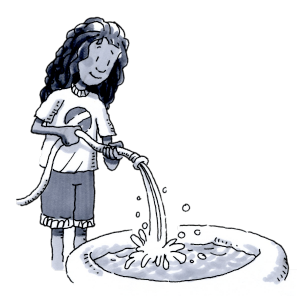### Home > CC2MN > Chapter 6 Unit 6B > Lesson CC2: 6.1.4 > Problem6-47

6-47.Beth is filling a small backyard pool with a garden hose. The pool holds $30$ gallons of water. After $5$ minutes, the pool is about one fourth full. Homework Help ✎

1. Assuming that the water is flowing at a constant rate, about how much water is going into the pool each minute?

First, find how many gallons one-fourth of the pool is.

$\frac{30 \text{Gallons}}{4}=7.5\text{ Gallons}$

To find the rate per minute, divide the amount by the time.

$\frac{7.5\text{ Gallons}}{5\text{ Minutes}}$

$1.5\frac{\text{Gallons}}{\text{Minute}}$

2. About how long will it take to fill the pool?

If one fourth of the pool is filled in $5$ minutes, how long would it take for four fourths (one whole) of the pool to be filled?